﻿ 透水床面明渠湍流时空平均特征的大涡模拟与分析
«上一篇文章快速检索 高级检索

 力学学报2015, Vol. 47Issue (5): 713-721  DOI: 10.6052/0459-1879-14-3820

### 引用本文 [复制中英文]

[复制中文]
Han Xu, He Guojian, Fang Hongwei, Fu Songy. LARGE-EDDY SIMULATION AND DOUBLE-AVERAGING ANALYSIS OF OPEN-CHANNEL FLOW OVER A PERMEABLE BED[J]. Chinese Journal of Ship Research, 2015, 47(5): 713-721. DOI: 10.6052/0459-1879-14-382.
[复制英文]

### 文章历史

2014-12-01收稿
2015-07-08录用
2015-07-09网络版发表

1. 清华大学水利系, 水沙科学与水利水电工程国家重点实验室, 北京100084;
2. 清华大学工程力学系, 北京100084

1 双平均理论

 $\theta = \bar {\theta } + {\theta }'$ (1)
 $\bar {\theta } = \left\langle \bar {\theta } \right\rangle + \tilde {\theta }$ (2)

 $\left\langle {\bar \tau } \right\rangle = - \rho \left\langle {\tilde u\tilde w} \right\rangle - \rho \left\langle {\overline {u'w'} } \right\rangle + \rho v\frac{{{\rm{d}}\left\langle {\bar u} \right\rangle }}{{{\rm{d}}z}}$ (3)

2 数值模拟简介 2.1 控制方程组

 $\dfrac{\partial u_i }{\partial x_i } = 0$ (4)
 $\frac{{\partial {u_i}}}{{\partial t}} + \frac{\partial }{{\partial {x_j}}}\left( {{u_i}{u_j}} \right) = {g_i} - \frac{1}{\rho }\frac{{\partial p}}{{\partial {x_i}}} + \nu \frac{{{\partial ^2}{u_i}}}{{\partial x_j^2}}{\rm{ }}$ (5)

 $p = p_{\rm s} + p_{\rm d}$ (6)

 ${g_i} = \frac{1}{\rho }\frac{{\partial {p_{\rm{s}}}}}{{\partial {x_i}}}$ (7)

 $g_i - \dfrac{1}{\rho }\dfrac{\partial p}{\partial x_i } = \dfrac{1}{\rho }\dfrac{\partial p_{\rm s} }{\partial x_i } - \dfrac{1}{\rho }\dfrac{\partial \left( {p_{\rm s} + p_{\rm d} } \right)}{\partial x_i } = - \dfrac{1}{\rho }\dfrac{\partial p_{\rm d} }{\partial x_i }$ (8)

 $\dfrac{\partial \bar {u}_i }{\partial x_i } = 0$ (9)
 $\dfrac{\partial \bar {u}_i }{\partial t} + \dfrac{\partial }{\partial x_j }\left( {\overline {u_i u_j } } \right) = \dfrac{1}{Re}\dfrac{\partial ^2\bar {u}_i }{\partial x_j^2 } - \dfrac{\partial \bar {p}}{\partial x_i }$ (10)

 $- \overline {{u}'_i {u}'_j } = 2\nu _t \bar {S}_{ij} - \dfrac{1}{3}\delta _{ij} \overline {{u}'_k {u}'_k }$ (11)

2.2 数值模拟参数设置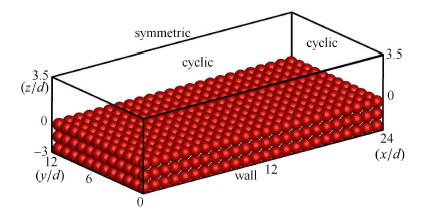图 1 模拟河床示意 Fig.1 Variation of roughness geometry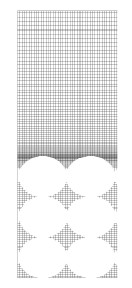图 2 数值网格示意 Fig.2 The numerical grid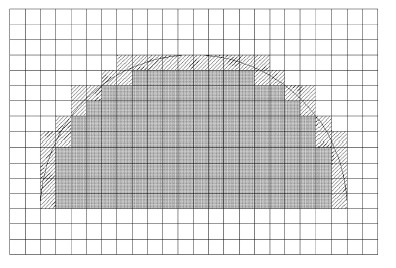图 3 浸没边界法不同类型网格示意图(灰色为固体网格，阴影为边界网格，白色为流体网格) Fig.3 Schematic of different types of grids in IBM (solid grids:girds filled with grey,boundary grids: grids filled with slashes，flow grids:grids filled with white)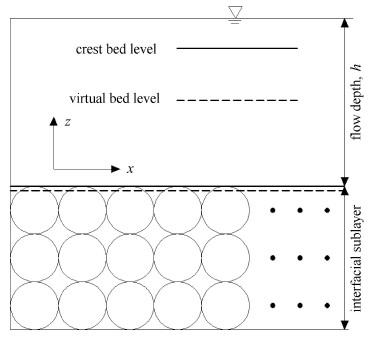图 4 流层示意 Fig.4 Illustration of the flow sublayers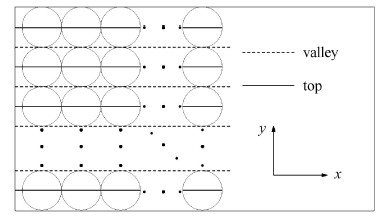图 5 取样位置示意 Fig.5 Illustration of the sample position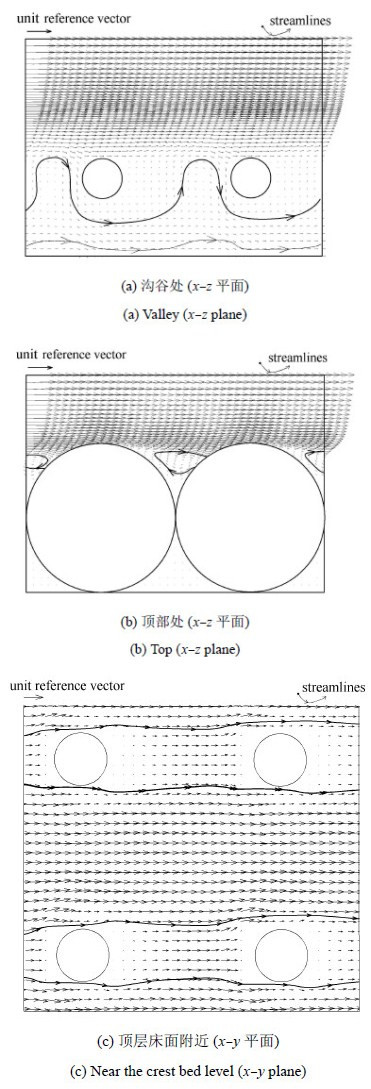图 6 沟谷与顶部的时均流速矢量和流线图 Fig.6 Time-averaged velocity vectors and streamlines
3 时空双平均湍流分析 3.1 时空双平均流速

 $u^ + = \dfrac{1}{\kappa }\ln \dfrac{z_1 ^ + + z_0 ^ + }{k_{\rm s}^ + } + B$ (12)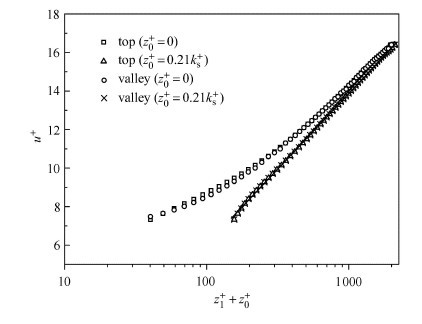图 7 平均床面修正前后的流速分布 Fig.7 Velocity distribution with and without the modification of theoretic

 $u^ + = \dfrac{1}{0.292}\ln \dfrac{z_1 ^ + + 0.21k_{\rm s}^ + }{k_{\rm s} ^ + } - 9.76$ (13)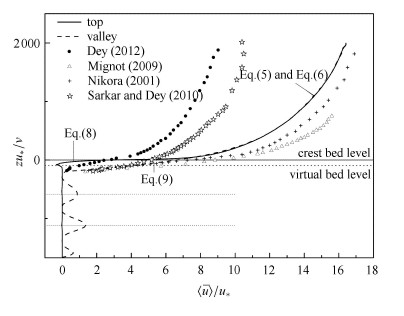图 8 双平均顺流流速垂向分布 Fig.8 DA streamwise velocity profiles obtained from LES and data points of previous experimental studies

 $u^ + = \dfrac{1}{0.286}\ln \dfrac{z_1 ^ + + 0.21k_{\rm s}^ + }{k_{\rm s}^ + } - 10.232$ (14)

 $u^ + = \dfrac{1}{0.28}\ln \dfrac{z + 0.32}{D_{50} } + 8.21$ (15)

 $z_1 ^ + = 5.823\,3u^ {+ 3} - 31.939u^{ + 2} + 64.839u^ + - 57.312$ (16)

 $z_1 ^ + = 36.545u^ + - 244.05$ (17)

3.2 时空双平均总剪应力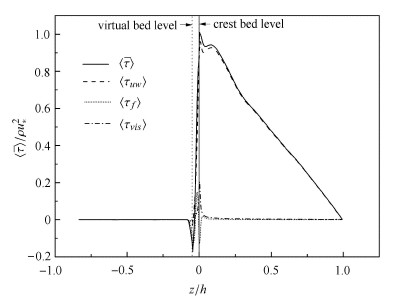图 9 双平均剪应力垂向分布 Fig.9 DA fluid shear stress
3.3 时空双平均雷诺剪应力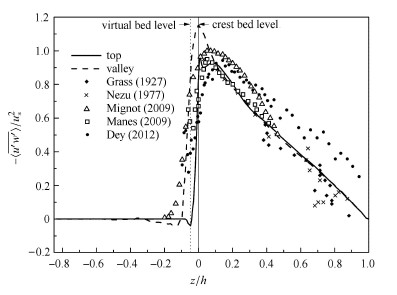图 10 顶部及沟谷双平均雷诺剪应力 Fig.10 DA Reynolds shear stress
3.4 时空双平均构造剪应力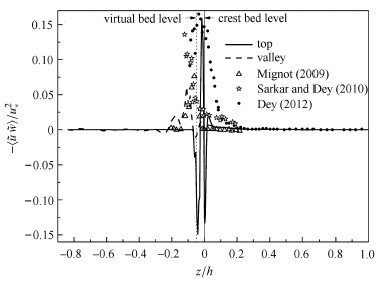图 11 顶部及沟谷双平均构造剪应力 Fig.11 DA Form-induced shear stress
3.5 时空双平均脉动幅度

 ${\sqrt {\overline {{u}'{u}'} } } / {u^\ast }$ (18)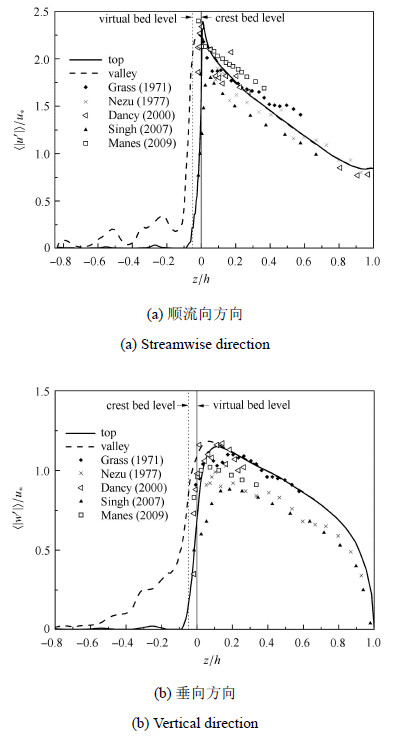图 12 双平均脉动幅度的垂向分布 Fig.12 DA Reynolds turbulence intensities

4 结 论

(1)理想粗糙透水床面明渠湍流，顶层床面上部流场中，即$z_1 ^ + > 80$范围内，双平均流速分布符合对数分布，且基本不受空间异构性影响. 但由于透水率较大，卡门常数变小，为0.29左右；

(2)靠近顶层床面，即$- 98 ＜ z_1 ^ + ＜ 80$范围内，由于空间异构性增强，不同位置的双平均流速分布出现了差异. 其中，顶部双平均流速分布符合多项式分布，沟谷双平均流速分布符合线性分布；

(3)透水床面内部，靠近底面球孔双平均流速约为靠近上部球孔双平均流速的1.55倍.

(4)在顶层床面之上，即$z/h> 0.01$时，雷诺剪应力占有主体地位，大约为总应力的95%. 靠近顶层床面，即$- 0.1 ＜ z/h ＜ 0.01$时，由于紊动增强，空间异构性增大，构造剪应力不能忽略，约占总应力的15%.

(5)由于空间异构性，在靠近顶层床面时，不同空间位置的剪应力及湍流脉动幅度峰值大小及峰值出现的位置有所不同.

  Smith JD, Mclean SR. Spatially averaged flow over a wavy surface. Journal of Geophysical Research-Oceans and Atmospheres, 1977, 82(12): 1735-1746  Nikora V, Koll K, Mcewan I, et al. Velocity distribution in the roughness layer of rough-bed flows. Journal of Hydraulic Engineering-ASCE, 2004, 130(10): 1036-1042  Coleman SE, Nikora VI, Mclean SR, et al. Subelement form-drag parameterization in rough-bed flows. Journal of Hydraulic Engineering-ASCE, 2007, 133(2): 121-129  Aberle J. Measurements of armour layer roughness geometry function and porosity. Acta Geophysica, 2007, 55(1): 23-32  Dey S, Das R. Gravel-Bed hydrodynamics: Double-averaging approach. Journal of Hydraulic Engineering-ASCE, 2012, 138(8): 707-725  Mignot E, Hurther D, Barthelemy E. On the structure of shear stress and turbulent kinetic energy flux across the roughness layer of a gravel-bed channel flow. Journal of Fluid Mechanics, 2009, 638: 423-452  Jimenez J. Turbulent flows over rough walls. Annual Review of Fluid Mechanics, 2004, 36: 173-196  白静,方红卫,何国建. 非淹没丁坝绕流的三维大涡模拟研究. 力学学报, 2013, 45(2): 151-157 (Bai Jing, Fang Hongwei, He Guojian. Study of non-submerged groin turbulence flowin a shallowopen channel by LES. Chinese Journal of Theoretical and Applied Mechanics, 2013, 45(2): 151-157 (in Chinese))  Nikora VI, Smart GM. Turbulence characteristics of New Zealand gravel-bed rivers. Journal of Hydraulic Engineering-ASCE, 1997, 123(9): 764-773  Kironoto BA, Graf WH. Turbulence characteristics in rough uniform open-channel flow. Proceedings of the Institution of Civil Engineers-Water Maritime and Energy, 1994, 106(4): 333-344  Nikora VI, Rowinski PM. Rough-bed flows in geophysical, environmental, and engineering systems: Double-averaging approach and its applications - preface. Acta Geophysica, 2008, 56(3): 529-533  Gimenez-Curto LA, Corniero MA. Flow characteristics in the interfacial shear layer between a fluid and a granular bed. Journal of Geophysical Research-Oceans, 2002, 107(C5): 12-1-12-9  Nikora V, Mcewan I, Mclean S, et al. Double-Averaging concept for rough-bed open-channel and overland flows: Theoretical background. Journal of Hydraulic Engineering-ASCE, 2007, 133(8): 873-883  Manes C, Pokrajac D, Mcewan I. Double-averaged open-channel flows with small relative submergence. Journal of Hydraulic Engineering-ASCE, 2007, 133(8): 896-904  Nikora V, Goring D, Mcewan I, et al. Spatially averaged open-channel flow over rough bed. Journal of Hydraulic Engineering-ASCE, 2001, 127(2): 123-133  Germano M, Piomelli U, Moin P, et al. A dynamic subgrid-scale eddy viscosity model. Physics of Fluids A-Fluid Dynamics, 1991, 3(7): 1760-1765  Bomminayuni S, Stoesser T. Turbulence statistics in an open-channel flow over a rough bed. Journal of Hydraulic Engineering-ASCE, 2011, 137(11): 1347-1358  Dasgupta A, Paudyal GN. Characteristics of free-surface flow over gravel bed. Journal of Irrigation and Drainage Engineering-ASCE, 1985, 111(4): 299-318  陈兴伟,林木生,程年生等. 粗糙透水床面明渠水流的垂线流速分布. 水科学进展, 2013, 24(6): 849-854 (Chen Xingwei, Lin Musheng1, Cheng Niansheng, et al. Velocity profile of turbulent open-channel flows over rough and permeable beds. Advances in Water Science, 2013, 24(6): 849-854 (in Chinese))  Nezu I, Kadota A, Nakagawa H. Turbulent structure in unsteady depth-varying open-channel flows. Journal of Hydraulic Engineering-ASCE, 1997, 123(9): 752-763  Manes C, Pokrajac D, Nikora VI, et al. Turbulent friction in flows over permeable walls. Geophysical Research Letters, 2011, 38: L03402  Manes C, Pokrajac D, Mcewan I, et al. Turbulence structure of open channel flows over permeable and impermeable beds: A comparative study. Physics of Fluids, 2009, 21: 12510912  Nezu I, Nakagawa H. Turbulence in Open-Channel Flows. Balkema, Rotterdam, Netherlands. 1993
LARGE-EDDY SIMULATION AND DOUBLE-AVERAGING ANALYSIS OF OPEN-CHANNEL FLOW OVER A PERMEABLE BED
Han Xu, He Guojian, Fang Hongwei, Fu Song
1. Department of Hydraulic Engineering, Tsinghua University, The State Key Laboratory of Hydro Science and Engineering, Beijing 100084, China;
2. School of Aerospace, Tsinghua University, Beijing 100084, China
Fund: The project was supported by the National Natural Science Foundation of China (11372161) and the State Key Laboratory of Hydro-Science and Engineering, MOST, China (2014-KY-02).
Abstract: This study focuses on the double-averaging (DA) turbulence characteristics of flow over an idealized permeable bed using the large-eddy simulation (LES). To discuss the spatial heterogeneity, the vertical distributions of DA velocity, DA Reynold shear stress (RSS), DA form-induced shear stress (FISS) and turbulence intensities in different positions are analyzed. It is shown that the spatial heterogeneity has a little influence in the upper flow region, and the distribution of DA velocity corresponds to the logarithmic law. The Von Karman constant obtained is significantly less than the normal value because of permeability. In the vicinity of bed, the spatial heterogeneity has a significant effect on the velocity distributions, which match with a polynomial series and a linear series respectively. Within the permeable bed, the peak velocity at lower pore is 1.55 times larger than that at upper pore. Above the crest bed level, the DA Reynolds shear stress is 95% of the total DA shear stress. The DA form-induced shear stress is 15% of the total DA shear stress occurring at the virtual bed level. The distributions of turbulence intensities in different positions show some discrepancies, which result from the spatial heterogeneity.
Key words: turbulence    large-eddy simulation    double-averaged method    permeable bed